Homework Help Question & Answers

# B only It’s review question, I need this as soon as possible. Thank you 3) Pol the diferential equation: (a) The point o -1 is an ordinary point. Compute the recursion formula for the coeffici...

B only

It’s review question, I need this as soon as possible. Thank you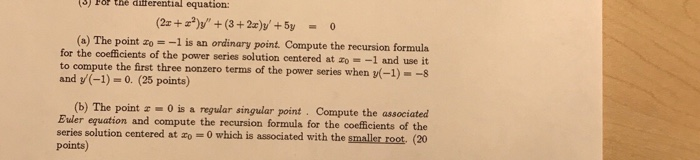3) Pol the diferential equation: (a) The point o -1 is an ordinary point. Compute the recursion formula for the coefficients of the power series solution centered at zo- -1 and use it to compute the first three nonzero terms of the power series when (-1)--s and y(-1)-0. (25 points) (b) The point 0 is a regular singular point Compute the associated Buler equation and compute the recursion formula for the coefficients of the series solution centered at o 0 which is associated with the smaller root. (20 points)

#### Homework Answers

Answer #1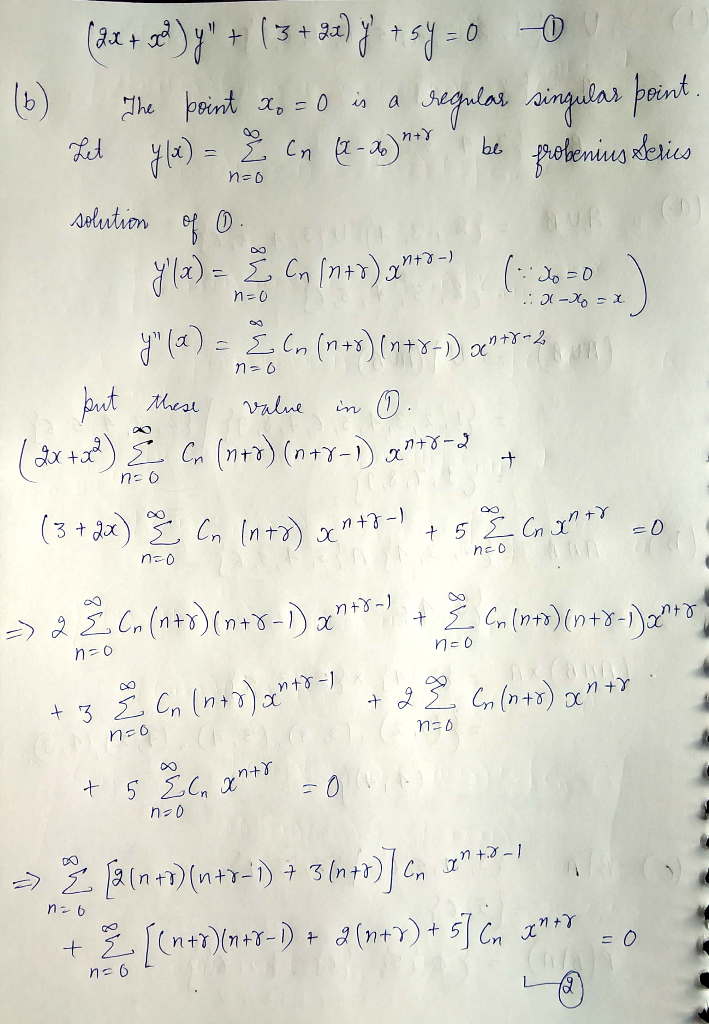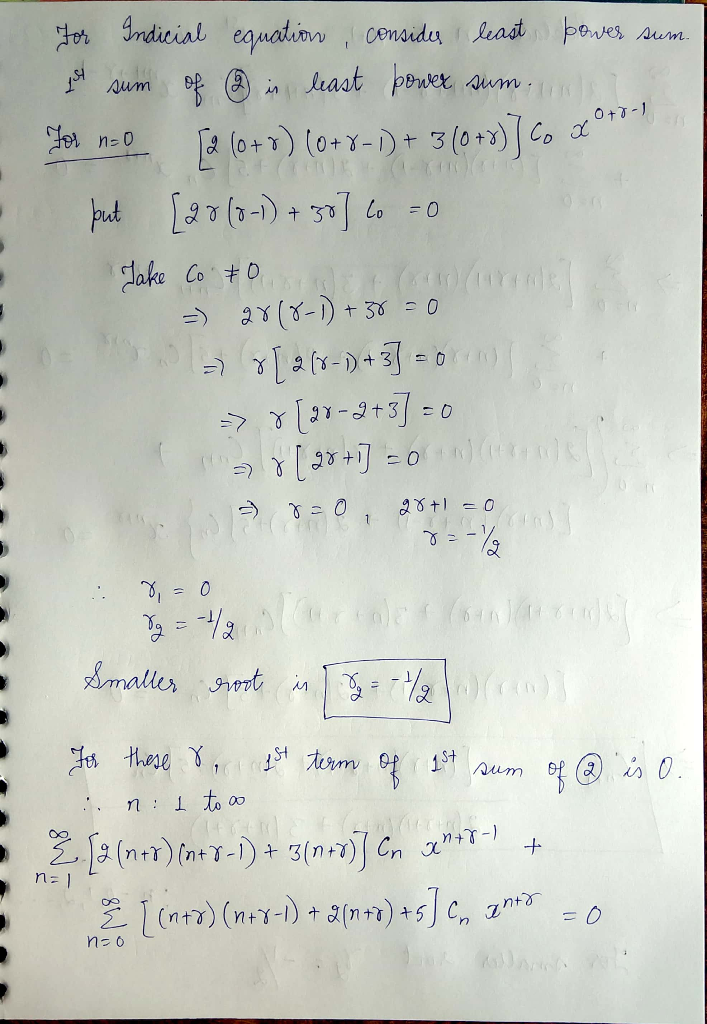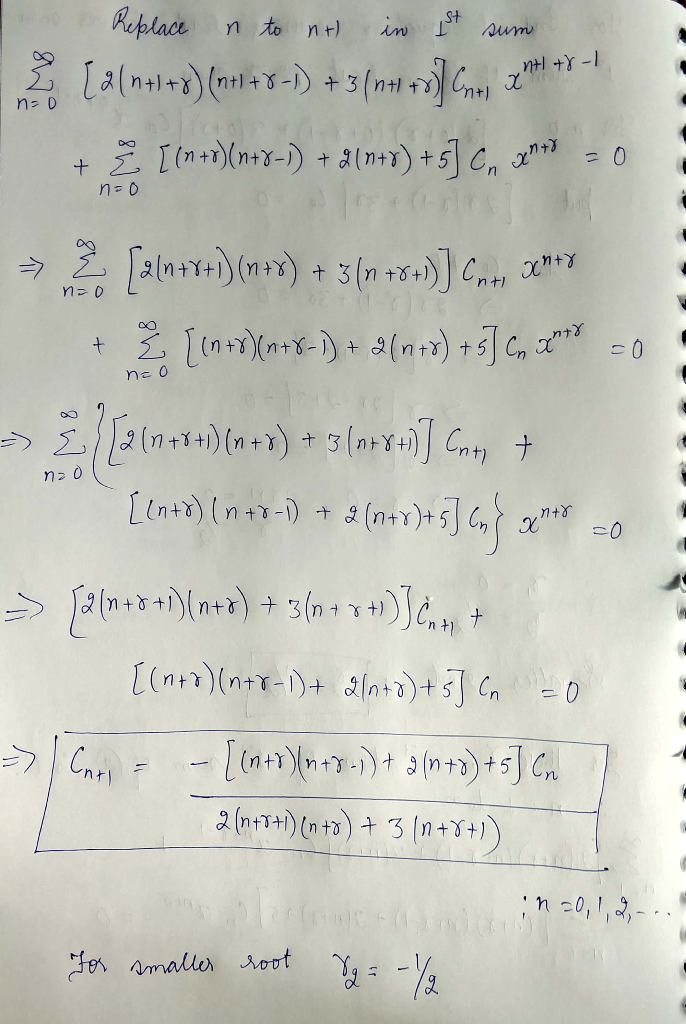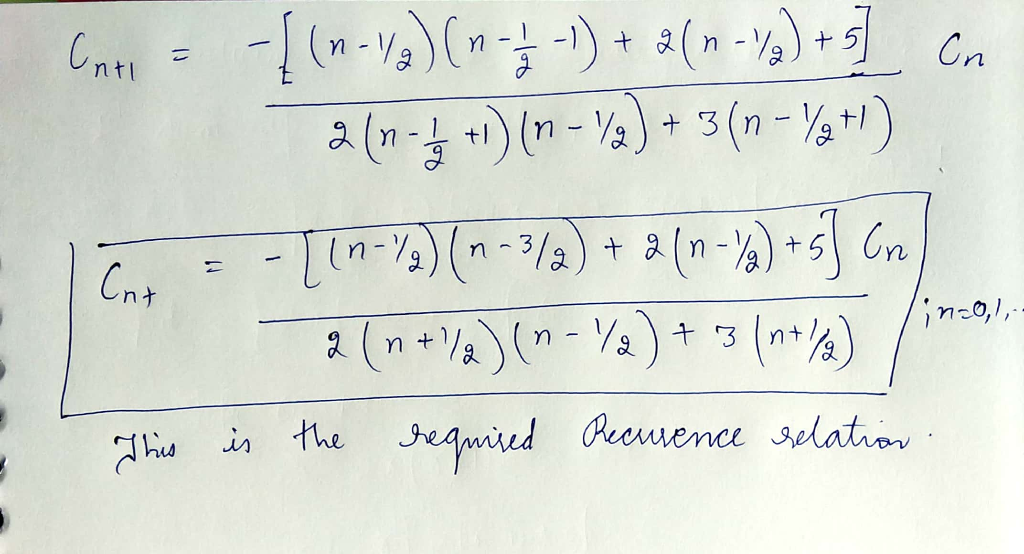Know the answer?
Your Answer:

#### Post as a guest

Your Name:

What's your source?

#### Earn Coin

Coins can be redeemed for fabulous gifts.

Not the answer you're looking for? Ask your own homework help question. Our experts will answer your question WITHIN MINUTES for Free.
Similar Homework Help Questions
• ### 3) Pol the diferential equation: (a) The point o -1 is an ordinary point. Compute the recursion formula for the coefficients of the power series solution centered at zo- -1 and use it to compute...3) Pol the diferential equation: (a) The point o -1 is an ordinary point. Compute the recursion formula for the coefficients of the power series solution centered at zo- -1 and use it to compute the first three nonzero terms of the power series when (-1)--s and y(-1)-0. (25 points) (b) The point 0 is a regular singular point Compute the associated Buler equation and compute the recursion formula for the coefficients of the series solution centered at o 0...

• ### It’s review question, I need this as soon as possible. Thank you 3) For thè diferential equation: (a) The point zo =-1 is an ordinary point. Compute the recursion formula for the coefficients of...It’s review question, I need this as soon as possible. Thank you3) For thè diferential equation: (a) The point zo =-1 is an ordinary point. Compute the recursion formula for the coefficients of the power series solution centered at zo- -1 and use it to compute the first three nonzero terms of the power series when -1)-s and v(-1)-0. (25 points) (b) The point z = 0 is a regular singular point . Compute the associated Euler equation and compute...

• ### (6) (18 points) For the differential equation: Compute the recursion formula for the coefficients...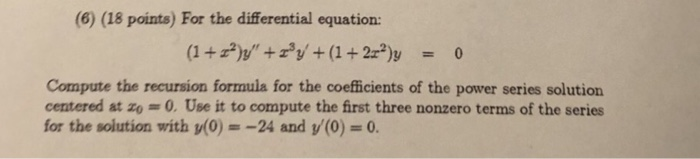(6) (18 points) For the differential equation: Compute the recursion formula for the coefficients of the power series solution centered at zo 0.Use it to compute the first three nonzero terms of the series for the solution with y(0) =-24 and y(0) = 0. (6) (18 points) For the differential equation: Compute the recursion formula for the coefficients of the power series solution centered at zo 0.Use it to compute the first three nonzero terms of the series for the...

• ### (4) (12 points)For the differential equation: Compute the recursion formula for the coefficients of the power series solution centered at o 0 and use it to compute the first three nonzero terms of...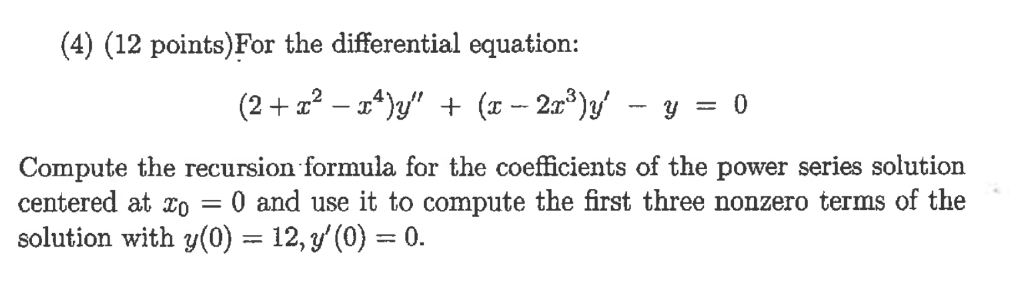(4) (12 points)For the differential equation: Compute the recursion formula for the coefficients of the power series solution centered at o 0 and use it to compute the first three nonzero terms of the solution with y(0) 12, y'(0)0. (5) (12 points)For the equation y" - 5ty -7y 0 (t>0), (t)t is a solution (a) Use the method of Reduction of Order to obtain a second, independent solution. (b) Solve the equation directly, using that it is an Euler Equation....

• ### Question 3 Consider the ordinary differential equation (ODE) 2xy" + (1 + x)y' + 3y =...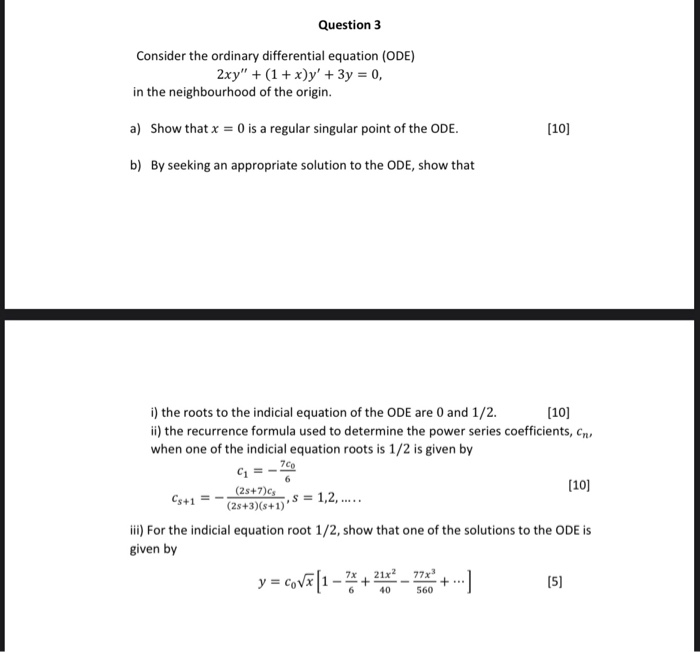Question 3 Consider the ordinary differential equation (ODE) 2xy" + (1 + x)y' + 3y = 0, in the neighbourhood of the origin. a) Show that x = 0 is a regular singular point of the ODE. (10) b) By seeking an appropriate solution to the ODE, show that G=- (10) i) the roots to the indicial equation of the ODE are 0 and 1/2.  ii) the recurrence formula used to determine the power series coefficients, ens when one...

• ### Question 2 (3 points) For the equation Ir-16"(x-ib"-20' +y-o, the point r-i is 2xy +y -0,...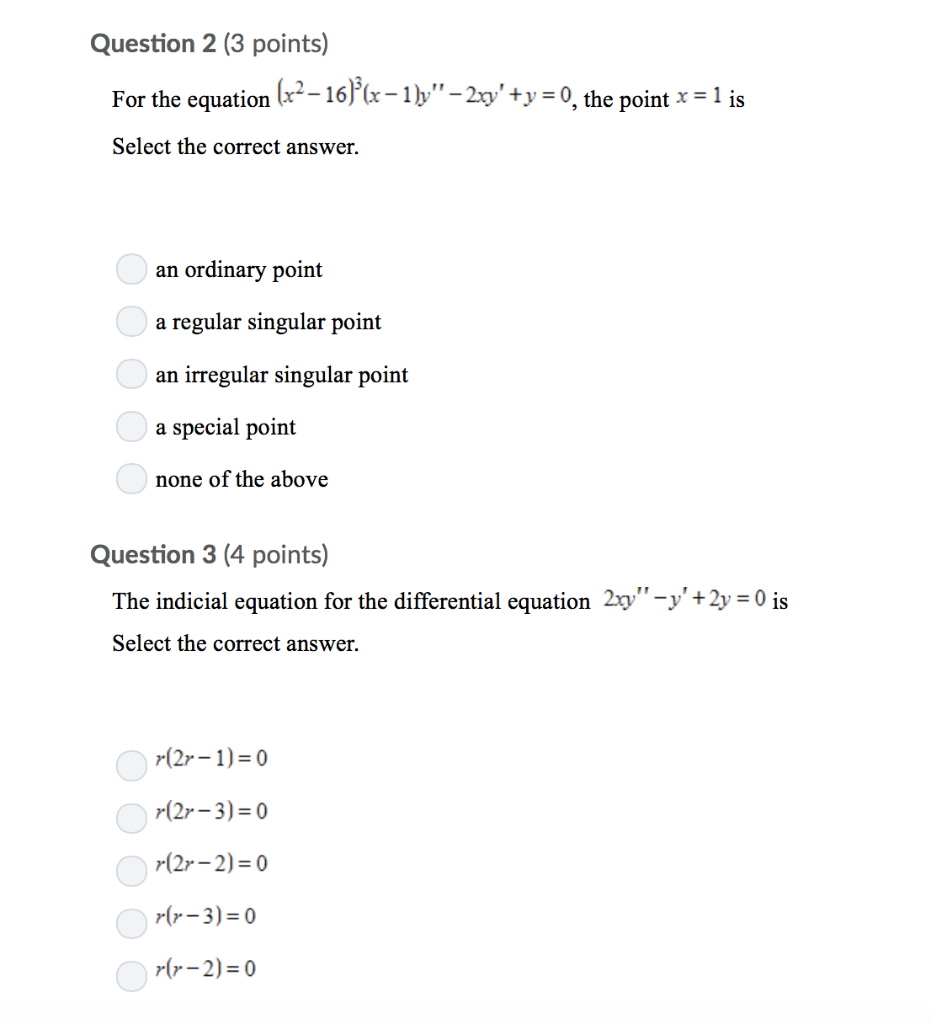Question 2 (3 points) For the equation Ir-16"(x-ib"-20' +y-o, the point r-i is 2xy +y -0, the point x-1 is Select the correct answer. an ordinary point a regular singular point an irregular singular point a special point none of the above Question 3 (4 points) The indicial equation for the differential equation 2xy-'+2y 0 is Select the correct answer. (2r-1)0 (2r-3) 0 (2r-2)-0 rr-3)-0 r(r-2)0

• ### Question 2 In this question you need to construct a homogeneous linear second order differential equations satisfying particular things . The DE has a regular singular point at 1 and an irregular sin...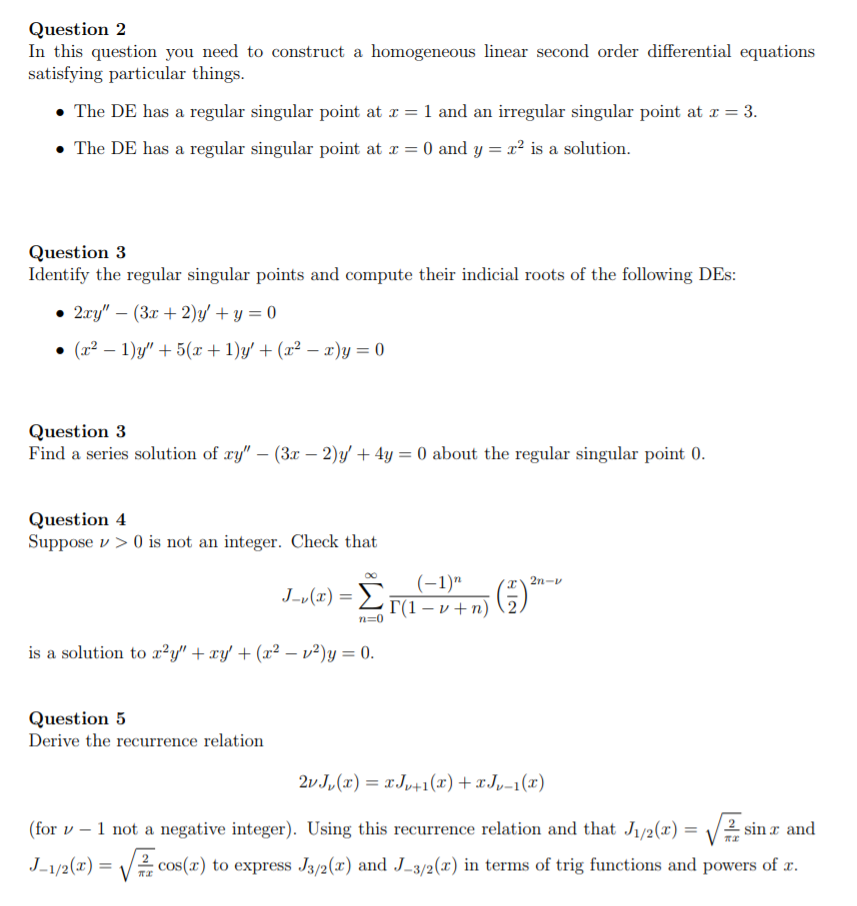Question 2 In this question you need to construct a homogeneous linear second order differential equations satisfying particular things . The DE has a regular singular point at 1 and an irregular singular point at 3 X2 Is a solution The DE has a regular singular point at x 0 and y Question 3 Identify the regular singular points and compute their indicial roots of the following DEs Question 3 Find a series solution of ry" - (3x - 2)y...

• ### 1) Find two power series solutions of the differential equation (x² + 1)y" – xy' +...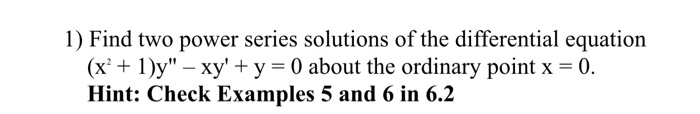1) Find two power series solutions of the differential equation (x² + 1)y" – xy' + y = 0 about the ordinary point x = 0. Hint: Check Examples 5 and 6 in 6.2 Example 6 Power Series Solution Solve (x + 1)," + xy - y = 0. Solution As we have already seen the given differential equation has singular points at = = ti, and so a power series solution centered at o will converge at least for...

• ### I need help with these! 3. (1 point) a) Compute the general solution of the differential equation y"5 12y" 0 b) Determine the test function Y (t) with the fewest terms to be used to obtain...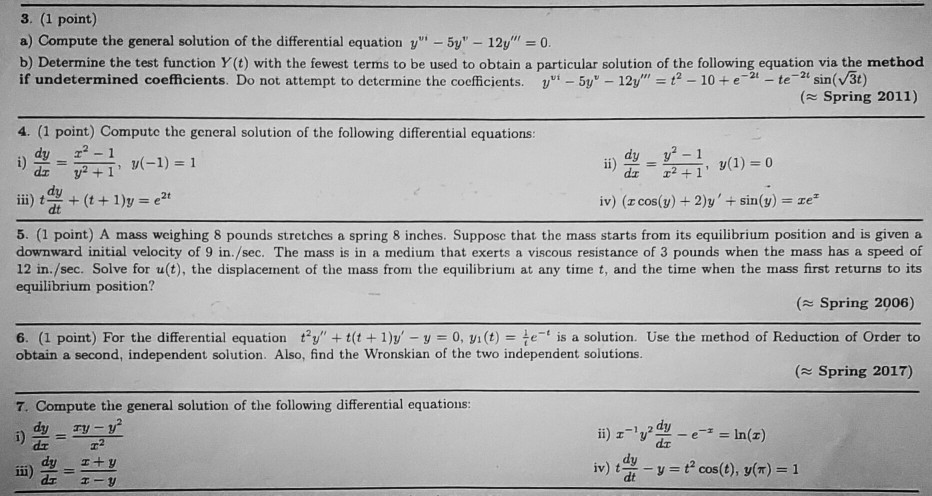I need help with these! 3. (1 point) a) Compute the general solution of the differential equation y"5 12y" 0 b) Determine the test function Y (t) with the fewest terms to be used to obtain a particular solution of the following equation via the method if undetermined coefficients. Do not attempt to determine the coefficients.5y 12y"2 10e-tesin(V3t) Spring 2011) 4. (1 point) Compute the general solution of the following differential equations dz dy dt ii)(1y iv) (z cos(y) +...

• ### (1 point) Frobenius' method: finding solutions as generalized power series Example: Consider the equation Tºg +...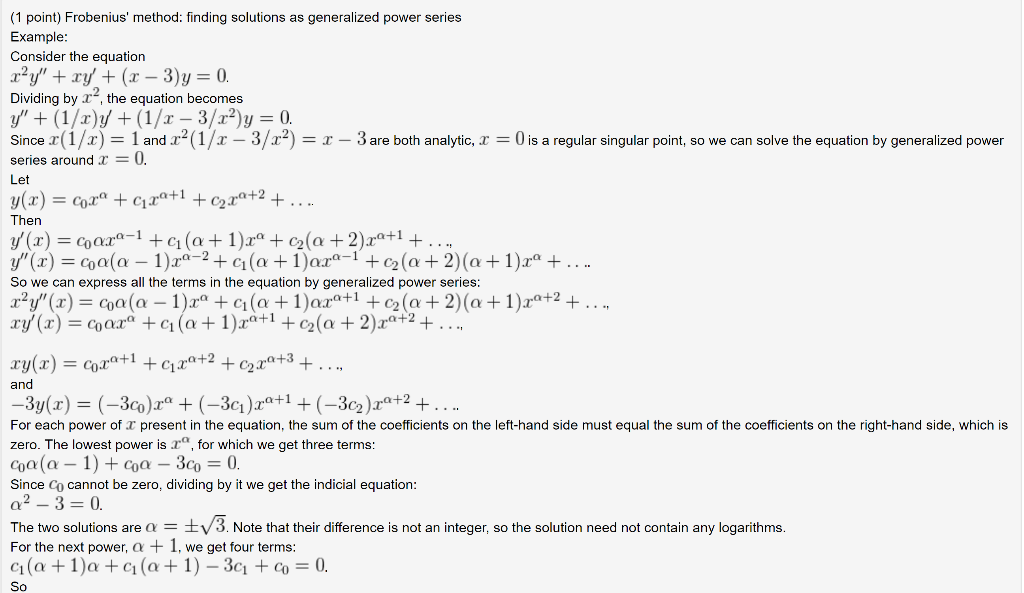(1 point) Frobenius' method: finding solutions as generalized power series Example: Consider the equation Tºg + Tự+(x - 3) = 0. Dividing by r, the equation becomes y' + (1/2y + (1/x - 3/x)y = 0. Sincer(1/) = 1 and .ca(1/x - 3/) = x - 3 are both analytic, x = 0 is a regular singular point, so we can solve the equation by generalized power series around x = 0. Let y(x) = Cox® + C1.+1 + c2r4+2...

Free Homework App

Scan Your Homework
to Get Instant Free Answers
Need Online Homework Help?

Get Answers For Free
Most questions answered within 3 hours.